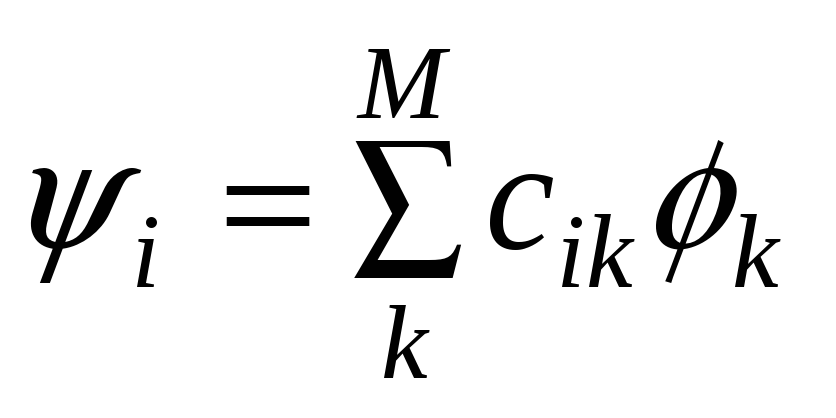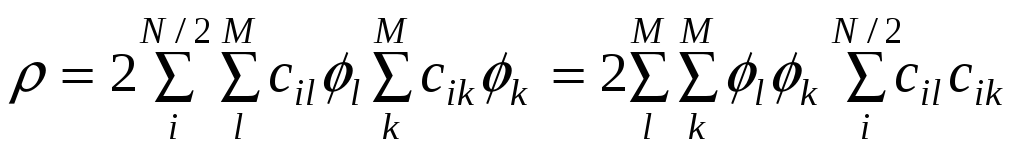# Bis(pentafluorophenyl)boryl ligand

 Page 1/4 Date 09.06.2018 Size 309.8 Kb.
1   2   3   4

Perfluoroaryl boryl complexes: synthesis, spectroscopic and structural characterisation of a complex containing the bis(pentafluorophenyl)boryl ligand.
S. Aldridge,*a A. Al-Fawaz,a R.J. Calder,a A.A. Dickinson,a D.J. Willock,a M.E. Light,b and M.B. Hursthouse.b
a Department of Chemistry, Cardiff University, PO Box 912, Park Place, Cardiff, UK CF10 3TB.

b EPSRC National Crystallography Service, Department of Chemistry, University of Southampton, Highfield, Southampton, UK SO17 1BJ.

## Supplementary Data

A. Computational methodology
The computational methodology used in the calculation of geometric and bonding parameters for compounds 2, 3 and 4 is detailed below and is very similar to that used in reference 7.
Gradient corrected DFT calculations were carried out using the ADF2000.01 code [E.J. Baerends, D.E. Ellis and P. Ros, Chem. Phys., 1973, 2, 41; L. Versluis and T. Ziegler, J. Chem. Phys., 1988, 88, 322; G. te Velde and E.J. Baerends, J Comput. Phys. 1992, 99, 84; C. Fonseca Guerra, J.G. Snijders, G. te Velde, and E.J. Baerends, Theor. Chem. Acc. 1998, 99, 391], with functionals for exchange and correlation due to Becke [A. D. Becke, Phys. Rev. A, 1988, 38, 3098] and Lee, Yang and Parr [C. Lee, W. Yang and R.G. Parr, Phys. Rev. B, 1988, 37, 785], respectively. A basis set constructed from Slater type orbitals at the triple zeta with polarization functions level was used for all calculations (ADF IV). The level of frozen core approximation for B, C, O and F was the 1s orbital and for Fe was the 2p orbital. All structures were fully optimised with no symmetry restrictions. Convergence was accepted when the following limits were met: (i) energy change on next step < 1x10-3 Ha; (ii) gradient < 1x10-3 Ha Å-1; and (iii) uncertainty in cartesian coordinates < 1x10-2 Å. The multiplicity of each structure was determined by using unrestricted calculations with spin states set to reasonable alternatives to determine the lowest energy configuration which conformed to the aufbau principle.
The bond dissociation energy for given MB bonds was calculated by estimating the energy change associated with the homolytic bond cleavage reaction:
(5-C5H5)Fe(CO)2(BX2)  (5-C5H5)Fe(CO)2 + BX2

The structures of the products from this reaction were independently optimised to allow for the inclusion of any molecular relaxation. The effect of basis set superposition error (BSSE) on the bond dissociation energy for compound 3 was calculated using the counterpoise method to be of the order of 7.5 kJ mol-1, a value comparable to that found for metal-carbonyl and metal-metal bonds using similar basis sets (6-8 kJ mol-1) [A. Rosa, A.W. Ehlers, E.J. Baerends, J.G. Snijders and G.; te Velde, J. Phys. Chem., 1996, 100, 5690]. The small magnitude of the BSSE suggests that the basis set being used is of high quality, in agreement with conclusions based on FeB bond length as a function of basis set [A.A. Dickinson, D.J. Willock, R.J. Calder and S. Aldridge, submitted for publication to Organometallics].

To calculate the degree of  and -bonding between the metal centre and boron atom the optimised structures were re-orientated so that the bond was aligned with the z-axis. A bonding analysis was then carried out following the approach outlined below to give contributions to the bonding density segregated according to the symmetry of the atomic orbitals involved.
The one electron wavefunctions,, used to represent the density in these DFT calculations are constructed in the usual manner as a linear combination of atomic basis functions,:(1)
whereis the coefficient of the kth basis function in the ith molecular orbital and there are a total of M basis functions. The density, , is then given by summation over the occupied orbitals of the one electron densities:(2)
Where N is the total number of electrons and we only consider the restricted spin paired situation for simplicity, extension to the spin unrestricted case is straightforward. The rearranged form of the density expression allows the calculation to be performed via the definition of two square matrices with the dimension M. The first is usually referred to as the density matrix,